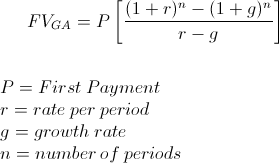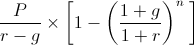# Future Value of Growing AnnuityThe formula for the future value of a growing annuity is used to calculate the future amount of a series of cash flows, or payments, that grow at a proportionate rate. A growing annuity may sometimes be referred to as an increasing annuity.

## Example of FV of Growing Annuity

An example of the future value of a growing annuity formula would be an individual who is paid biweekly and decides to save one of her extra paychecks per year. One of her net paychecks amounts to \$2,000 for the first year and she expects to receive a 5% raise on her net pay every year. For this example, we will use 5% on her net pay and not involve taxes and other adjustments in order to hold all other things constant. In an account that has a yield of 3% per year, she would like to calculate her savings balance after 5 years.

The growth rate in this example would be the 5% increase per year, the initial cash flow or payment would be \$2,000, the number of periods would be 5 years, and rate per period would be 3%. Using these variables in the future value of growing annuity formula would showAfter solving this equation, the amount after the 5th cash flow would be \$11,700.75.

## How is the FV of Growing Annuity Derived?

The future value of a growing annuity formula can be found by first looking at the following present value of a growing annuity formulaPresent Value can be converted into future value by multiplying the present value times (1+r)n. By multiplying the 2nd portion of the PV of growing annuity formula above by (1+r)n, the formula would show asFrom here, the formula above is the same as the formula shown at the top of the page after factoring out the initial payment, P.

New to Finance?